# Texas Go Math Grade 3 Lesson 12.5 Answer Key Divide by 4

Refer to our Texas Go Math Grade 3 Answer Key Pdf to score good marks in the exams. Test yourself by practicing the problems from Texas Go Math Grade 3 Lesson 12.5 Answer Key Divide by 4.

## Texas Go Math Grade 3 Lesson 12.5 Answer Key Divide by 4

Essential Question
What strategies can you use to divide by 4?

Unlock the Problem
A tree farmer plants 12 red maple trees in 4 equal rows. How many trees are in each row?

• What strategy could you use to solve the problem’
Answer: The strategy used is the division method to solve the problem

One Way
Make an array.

• Look at the array.
• Continue the array by drawing 1 tile in each of the 4 rows until all 12 tiles are drawn.
• Count the number of tiles in each row.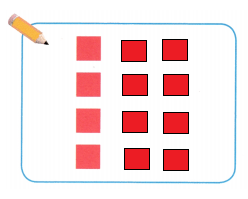There are _3__ tiles in each row.
So, there are _4_ trees in each row.
Write: _12__ ÷ _4__ = __3__ or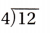Read: Twelve divided by four equals three.

Math Talk
Mathematical processes

Explain how making an array to solve the problem is like making equal groups.

Other Ways
A. Make equal groups.

• Draw 1 counter in each group.
• Continue drawing 1 counter at a time until all 12 counters are drawn.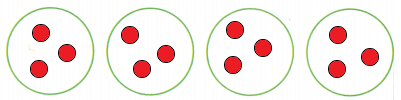There are _3_ counters in each group.

B. Use factors to find 12 ÷ 4.
The factors of 4 are 2 and 2.C. Use a related multiplication fact.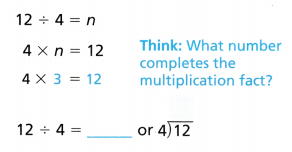Remember
A letter or symbol, like n, can stand for an unknown number.

Share and Show

Question 1.
Use the array to find 28 ÷ 4. ____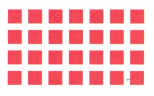Explanation:

Make an array.

• Look at the array.
• Continue the array by drawing 1 tile in each of the 4 rows until all 28 tiles are drawn.
• Count the number of tiles in each row.
There are _7_ tiles in each row.
And 4 rows and a total of 28 tiles are there.
Write: _28__ ÷ _4__ = __7__.

Math Talk
Mathematical Processes
Explain how you used the array to find the quotient.

Find the quotient.

Question 2.
___ = 21 ÷ 3
Explanation:Make an array.

• Look at the array.
• Continue the array by drawing 1 tile in each of the 3 rows until all 21 tiles are drawn.
• Count the number of tiles in each row.
There are _7_ tiles in each row.
And 3 rows and a total of 21 tiles are there.
Write: _21__ ÷ _3_ = __7__.

8 ÷ 4 = ____
Explanation: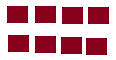Make an array.

• Look at the array.
• Continue the array by drawing 1 tile in each of the 2 rows until all 8 tiles are drawn.
• Count the number of tiles in each row.
There are 4 tiles in each row.
And 2 rows and a total of 8 tiles are there.
Write: _8__ ÷ _4_ = __2_.

Question 4.
___ = 40 ÷ 5
Explanation:Make an array.

• Look at the array.
• Continue the array by drawing 1 tile in each of the 5 rows until all 40 tiles are drawn.
• Count the number of tiles in each row.
There are 8 tiles in each row.
And 5 rows and a total of 40 tiles are there.
Write: _40__ ÷ _5_ = __8_.

Question 5.
24 ÷ 4 = ___
Explanation: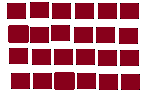Make an array.

• Look at the array.
• Continue the array by drawing 1 tile in each of the 4 rows until all 24 tiles are drawn.
• Count the number of tiles in each row.
There are 6 tiles in each row.
And 4 rows and a total of 24 tiles are there.
Write: _24_ ÷ _4_ = __6_.

Problem Solving

Practice: Copy and Solve Draw tiles to make an array.
Find the quotient.

Question 6.
30 ÷ 10
Explanation: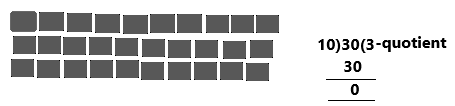Make an array.

• Look at the array.
• Continue the array by drawing 1 tile in each of the 3 rows until all 30 tiles are drawn.
• Count the number of tiles in each row.
There are 10 tiles in each row.
And 3 rows and a total of 30 tiles are there.
Write: _30_ ÷ _10_ = __3_.

Go Math Grade 3 Answer Key Pdf Divided By 4 Question 7.
15 ÷ 5
Explanation:Make an array.

• Look at the array.
• Continue the array by drawing 1 tile in each of the 3 rows until all 15 tiles are drawn.
• Count the number of tiles in each row.
There are 5 tiles in each row.
And 3 rows and a total of 15 tiles are there.
Write: _15_ ÷ _5_ = __3_.

Question 8.
40 ÷ 4
Explanation:Make an array.

• Look at the array.
• Continue the array by drawing 1 tile in each of the 4 rows until all 40 tiles are drawn.
• Count the number of tiles in each row.
There are 10 tiles in each row.
And 4 rows and a total of 40 tiles are there.
Write: _40_ ÷ _4_ = __10_.

Question 9.
16 ÷ 2
Explanation:Make an array.

• Look at the array.
• Continue the array by drawing 1 tile in each of the 2 rows until all 16 tiles are drawn.
• Count the number of tiles in each row.
There are 8 tiles in each row.
And 2 rows and a total of 16 tiles are there.
Write: _16_ ÷ _2_ = __8_.

Find the unknown number.

Question 10.
45 ÷ 5 = b
b= _9__
Explanation:
The factors of 5 are 1,5.
1*5=5
5*1=5
The factors of 45 are 9,5
5*9=45
9*5=45, these are the multiplication factors.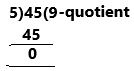Question 11.
20 ÷ 10 = e
e = _2__
Explanation:
The factors of 10 are 2,5
The factors of 20 are 2,4,5,10,20.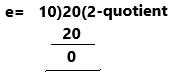Question 12.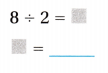Explanation: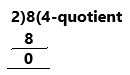divide 8 with 2then we get 4.

Texas Go Math Grade 3 Lesson 12.5 Answer Key Pdf Question 13.
24 ÷ 3 = h
h = __8_
The factors of 3 are 1,3
The factors of 24 are 3,8,1,24.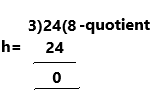Algebra Complete the table.

Question 14.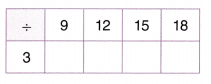Explanation:Question 15.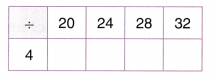Explanation: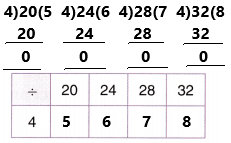H.O.T. Algebra Find the unknown number.

Question 16.
14 ÷ __ = 7
Explanation:
14÷n=7
Use a related multiplication factor
14=7*n
get 7 into the left side then 7 will be divided.
14÷7=n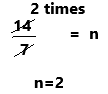2=n
Therefore, n=2

Question 17.
30 ÷ ___ = 6
Explanation:
30÷n=6
Use a related multiplication factor
30=6*n
get 6 into the left side then 6 will be divided.
30÷6=nn=5

Question 18.
8 ÷ ___ = 2
Explanation:
8÷n=2
Use a related multiplication factor
8=2*n
get 2 into the left side then 2 will be divided.
8÷2=nn=4

Question 19.
24 ÷ __ = 8
Explanation:
24÷n=8
Use a related multiplication factor
24=8*n
get 8 into the left side then 8 will be divided.
24÷8=n3=n

Problem Solving

Use the table for 20-22.

Question 20.
Derek planted dogwood trees in groups of 4. How many groups of trees did he plant?Explanation:
The number of dogwood trees Derek planted=24 {given in the table}
The number of groups he planted=4
The number of plants in each group=24÷4=6
Therefore, he planted 6 plants.

Multi-Step Douglas planted the birch trees in 4 equal rows. Then he added 2 more birch trees to each row. How many trees did he plant in each row?
Explanation:The number of birch trees Derek planted=16 {given in the table}
The number of groups he planted=4
After that, he added 2 more trees to each row=16+6=24
The number of plants in each group=24÷4=6
Therefore, he planted 6 plants.

Question 22.
H.O.T. Multi-Step Mrs. Banks planted the oak trees in 4 equal rows. Mr. Webb planted the dogwood trees in 3 equal rows. Who planted more trees in each row? How many more? Explain how you know.The number of oak trees Banks planted=28 {given in the table}
The number of groups he planted=4
The number of trees he planted in each group=28÷4=7
7 oak trees Banks planted in each row.
The number of dogwood trees Webb planted=24{given in the table}
The number of groups he planted=3
The number of trees he planted in each row=24÷3=8
8 dogwood trees were planted in each row by Webb.
Therefore, Webb planted more trees on each row.
Therefore, 8-7=1 more tree is there.

Question 23.
H.O.T. Write Math Bryan earns $40 mowing lawns each week. He earns the same amount of money for each lawn. If he mows 4 lawns, how much does Bryan earn for each lawn? Use Math Language to explain how you found your answer. Answer: 10 Explanation: The amount Bryan earns for mowing lawns each week=40$
The number of lawns he mows=4
The amount Bryan earn for each lawn=40/4=10
Therefore, 10$for each lawn. Daily Assessment Task Fill in the bubble for the correct answer choice. You may use models to help you solve. Question 24. Representations Jill has 12 shrimp. She puts an equal number of shrimp on 4 pizzas. How many shrimp does Jill put on each pizza? (A) 2 (B) 3 (C) 4 (D) 8 Answer: Option B is correct.Explanation: The number of shrimps Jill has=12 The number of shrimps she puts on pizza=4 The number of shrimps she puts on each pizza=12/4=3. Therefore, she puts 3 shrimps. Question 25. Ralph puts 36 chairs in 4 equal rows How many chairs does he put in each row? (A) 4 (B) 7 (C) 8 (D) 9 Answer: Option D is correct.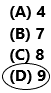Explanation: The number of chairs Ralph puts =36 The number of rows he puts equally=4 The number of chairs he puts in each row=36/4=9 Therefore, he puts 9 chairs. Question 26. Multi-Step Mia puts 15 animal stickers in 3 equal rows in her sticker hook She puts 28 flower stickers in 4 equal rows. How many more flower stickers than animal stickers are in each row? (A) 1 (B) 2 (C) 5 (D) 7 Answer: Option B is correct.Explanation: Total number of animal stickers Mia puts=15 Number of equal rows for animal stickers=3 Number of animal stickers in each row=15/3=5 Total number of flower stickers Mia puts=28 Number of equal rows for flower stickers=4 Number of flower stickers in each row=28/4=7 The number of more flower stickers than animal stickers are in each row=7-5=2. Texas Test Prep Go Math Lesson 12.5 3rd Grade Question 27. Aaron planted 16 tomato plants in 4 equal rows How many tomato plants are in each row? (A) 20 (B) 8 (C) 4 (D) 3 Answer: Option C is correct.Explanation: The number of tomato plants Aaron planted=16 The number of equal rows=4 The number of tomato plants in each row=16/4=4. Therefore, 4 plants in each row. ### Texas Go Math Grade 3 Lesson 12.5 Homework and Practice Answer Key Draw tiles to make an array. Find the quotient. Question 1. 16 ÷ 4 ____ Answer: 4 Explanation:Make an array. • Look at the array. • Continue the array by drawing 1 tile in each of the 4 rows until all 16 tiles are drawn. • Count the number of tiles in each row. There are 4 tiles in each row. And 4 rows and a total of 16 tiles are there. Write: _16_ ÷ _4_ = _4_. Question 2. 12 ÷ 6 = ___ Answer: 2 Explanation: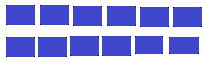Make an array. • Look at the array. • Continue the array by drawing 1 tile in each of the 2 rows until all 12 tiles are drawn. • Count the number of tiles in each row. There are 6 tiles in each row. And 2 rows and a total of 12 tiles are there. Write: 12 ÷ 6 = 2. Question 3. 15 ÷ 3 = ___ Answer: 5 Explanation:Make an array. • Look at the array. • Continue the array by drawing 1 tile in each of the 3 rows until all 15 tiles are drawn. • Count the number of tiles in each row. There are 5 tiles in each row. And 3 rows and a total of 15 tiles are there. Write: 15 ÷ 3 = 5. Problem Solving Use the table for 7-9. Question 7. Nate arranges the baskets of lettuce into 4 equal rows. How many baskets of lettuce are in each row?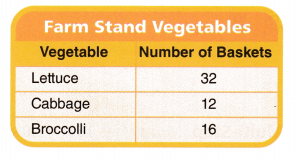Answer: 8 Explanation: The number of baskets of lettuce=32 { already given in the table} The number of rows he arranges =4 The number of lettuce in each row=32÷4=8 Therefore, 8 lettuce in each row. Question 8. Tia arranges the baskets of cabbage in 4 equal rows. She adds another basket to each row. How many baskets are in each row? Answer: 4 Explanation: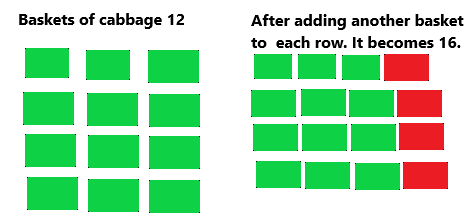The number of baskets of cabbage=12 The number of equal rows she arranged=4 Now, she adds another basket to every row=12+4=16 Now, The number of baskets in each row =16÷4=4. Therefore, 4 baskets in each row. Question 9. The farm stand gets 2 more baskets of broccoli. Erin wants to put them in equal rows of 4. Can she do this? Explain. Answer: No, she cannot, Explanation: Already we have 16 baskets of broccoli in the given table. Here, the farm stand gets another 2 baskets. so totally, 16+2=18. Now 18 baskets of broccoli are there. Here she wants to put in 4 equal rows. If we divide 18÷4=4.5. she can put more than 4 equal rows. But, here question asked was 4 equal rows. so she cannot place in 4 equal rows. Lesson Check Fill in the bubble completely to show your answer. Question 10. Mr. Benz arranges 24 music stands in class. He puts the stands in 4 equal rows. How many music stands are in each row? (A) 6 (B) 8 (C) 20 (D) 7 Answer: Option A is correct.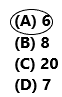Explanation: The number of music stands Benz arranges in a class=24 The number of equal rows=4 The number of music stands in each row=24÷4=6 Therefore, 6 stands in each row. Question 11. There are 36 members in a marching band. They march in 4 equal rows. How many band members are in each row? (A) 32 (B) 8 (C) 7 (D) 9 Answer: Option D is correct.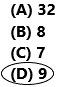Explanation: The number of members in a marching band=36 The number of equal rows in march=4 The number of band members in each row=36÷4=9 Therefore, 9 members in a row. Question 12. Evan spends$16 on 4 books. Each book costs the same amount. How much does each book cost?
(A) $12 (B)$4
(C) $5 (D)$6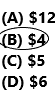Explanation:
The amount Evan spends on books=16$The number of books she spends=4 The cost of each book=16÷4=4$
Therefore, she spends 4\$ on each book.

Question 13.
There are 20 children at a party. The children are seated in equal groups at 4 tables. How many children are at each table?
(A) 24
(B) 6
(C) 5
(D) 16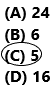Explanation:
The total number of children at a party=20
The number of children seated in equal groups=4
The number of children is at each table=20÷4=5.
Therefore, 5 children at each table.

Go Math Grade 3 Practice and Homework Lesson 12.5 Answer Key Question 14.
Multi-Step Monty has 16 toy cars in 4 equal groups and 24 toy boats in 3 equal groups. How many more toy boats are in each group than toy cars?
(A) 8
(B) 12
(C) 4
(D) 6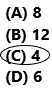Explanation:
The number of toys cars Monty has=16
The number of equal groups for cars=4
The number of toys boats Monty has=24
The number of equal groups for boats=3
The number of toys cars in each group=16÷4=4
The number of toys boats in each group=24÷3=8
The number of more toy boats are in each group than toy cars=8-4=4.
Therefore, 4 more toy boats are present.
Question 15.
Multi-Step Debra has 24 books in 4 equal piles and 20 magazines in 4 equal piles. If you stacked a pile of magazines on a pile of books, how many are in the pile?
(A) 6
(B) 7
(C) 8
(D) 11Explanation:
Total number of books Debra have in a pile=24
The number of equal piles for books=4
Total number of magazines Debra have in a pile=20
The number of equal piles for magazines=4
Now we need to find the number of books in each pile=24÷4=6
The number of magazines in each pile=20÷4=5
If she stacked a pile of magazines on a pile of books, then the number of total items in a pile could be=6+5=11.
Therefore, 11 items in a pile because there are 6 books and 5 magazines in each pile.

Scroll to Top